# Physical Chemistry : Valence Electrons

## Example Questions

### Example Question #1 : Valence Electrons

How many valence electrons are in an atom of phosphorus?

Eight

Three

Five

Thirteen

Five

Explanation:

When determining the number of valence electrons for an atom, simply count the number of electrons present in the outermost shell's s and p orbitals. Phosphorus has two electrons in the 3s subshell, and three more in the 3p subshell, making a total of five valence electrons.

### Example Question #2 : Valence Electrons

What is the complete electron configuration for the sulfur atom?

1s22s22p63s23p4

1s42p63s23p4

1s22s22p63s23p2

1s22s22p63s23p2

1s42p63s23p4

1s22s22p63s23p4

Explanation:

The types of subshells, from smallest to largest, are as follows: s, p, d, and f. These four subshells correspond respectively to the following quantum numbers: 0, 1, 2, and 3. From the periodic table, it is known that sulfur has 16 electrons. Additionally, the maximum number of electrons the s sublevel can hold is 2. The maximum number of electrons that the p subshell can hold is 6, and electrons fill orbitals from lowest to highest energy. 1s22s22p63s23p4 is the only choice that meets the criteria.

### Example Question #1 : Valence Electrons

Arrange the following ions in order of decreasing ionic radius: nitride ion, oxide ion, sodium ion, aluminum ion.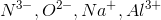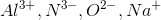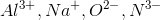The ions have the same ionic radius.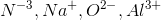Notice that all the ions have the same electron configuration as neon: 1s22s22p6Substances with the same number of electrons and the same electron configuration are isoelectronic, meaning the number of electrons is the same but the number of protons is not. In an isoelectronic series, the ion with the most protons is smallest because the nucleus exerts a stronger force of attraction and the electrons are pulled closer to the nucleus. Consequently, the ion with the fewest protons is largest.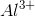has the most protons, therefore it has the smallest ionic radius. Continuing with this inverse relationship, the correct answer is:.